## Introduction

An algebraic expression is a mathematical statement containing variables. Examples: 2x, 7p2 – 3p + 2, 4m3 + 6n – ln, etc.

Every value of a variable is not a solution of an equation. Only a few values of variables will be the solutions of the equation, usually. But there are some equalities (for example, (x + y)2 = (x2 + 2xy + y2) which are true for any value of the variables involved in it. Such an equality is called an identity.

Rahul and Ankita are trying to find the product of two numbers. Ankita uses a different method and finds the product faster. Let us watch this video to know which method she used.

In the video, we understood that the product of two numbers can be found easily using identities.

Identities can also be used to find the square of a number.

#### Concepts

The chapter ‘Algebraic Expressions and Identities’ covers the following concepts:

#### Multiplication Of Algebraic Expressions

We know that the area of a rectangle is given by the product of the lengths of its two adjacent sides. A rectangle has two adjacent sides measuring ‘a’ units and ‘b’ units.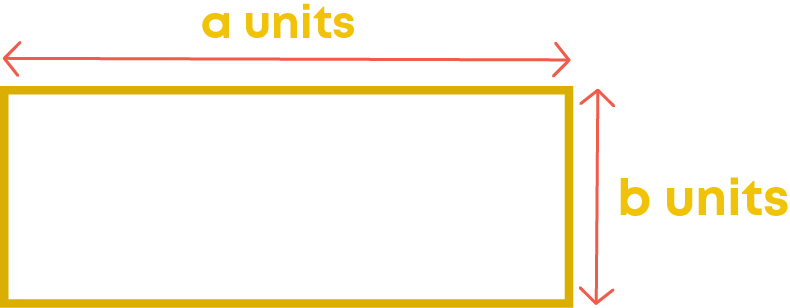How do you find its area?Here, ‘a’ and ‘b’ can take any value. That means they are variables. So, they can also be considered as algebraic expressions. What is the operation between ‘a’ and ‘b’ here? It is multiplication. So, the formula for the area of a rectangle is obtained by multiplying an algebraic expression by another. Thus, we use multiplication of algebraic expressions in many situations.

Multiplication of algebraic expressions is used to represent the total number of objects that can be arranged in rows and columns.

Multiplying A Monomial By A Monomial

To multiple the monomial 3 by the monomial 4x, the numerical coefficient of the first monomial is multiplied by the coefficient of the second monomial.Consider the multiplication of 2p by 3y. We know how to express the terms as the product of its factors. Can we say that monomials are same as terms? Yes, since a monomial has only one term, it can be called as a term.

Now, let us express the terms 2p and 3y as the products of their respective factors.So, 2p × 3y = 2 × p × 3 × y

Can you apply any law of numbers here to get the numbers together? Did you think of the commutative law? Yes, we can apply commutative law to get the numbers together.Now, we simplify it.

2p × 3y = 2 × 3 × p × y

= 6 × p × y

= 6py

Do you observe here that the numerical coefficient of one monomial is multiplied by that of the other and variable of one is multiplied by that of the other?Thus, while multiplying a monomial by another,

• The numerical coefficient of one gets multiplied by the numerical coefficient of the other.
• Variables of one are multiplied by that of the other.

Now, let us multiply 5p by 6py:You know that p × p = p2

Hence, 5p × 6py = 30p2y

Multiplication of variables follows the laws of exponents. For example,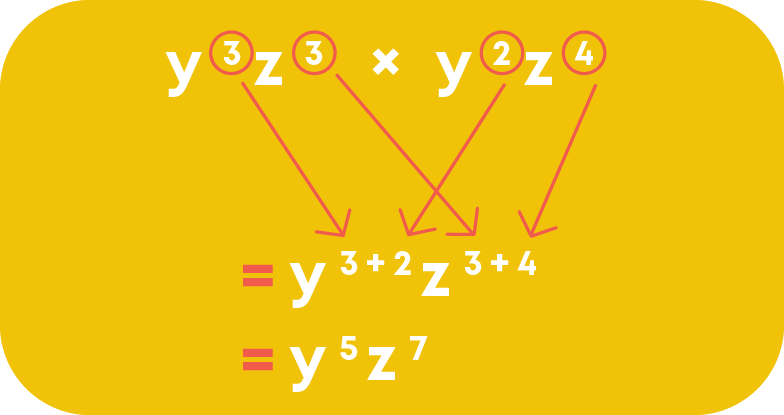Remember,

• The coefficient of the product of three or more monomials is the product of the coefficients of the monomials.
• The variables of the product of three or more monomials is the product of the variables of the monomials.

Example: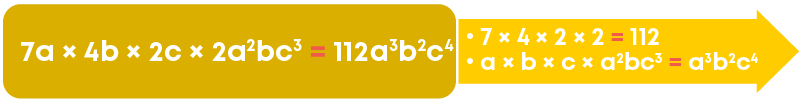Multiplying A Monomial By A Polynomial

We have learnt distributive property of numbers which states that if a, b, and c are three whole numbers, then

a × (b + c) = (a × b) + (a × c)

The distributive property tells you how to multiply a number by two or more numbers added inside brackets. It says that you can remove the brackets in this expression by multiplying the number outside the brackets by each number inside the brackets. Example,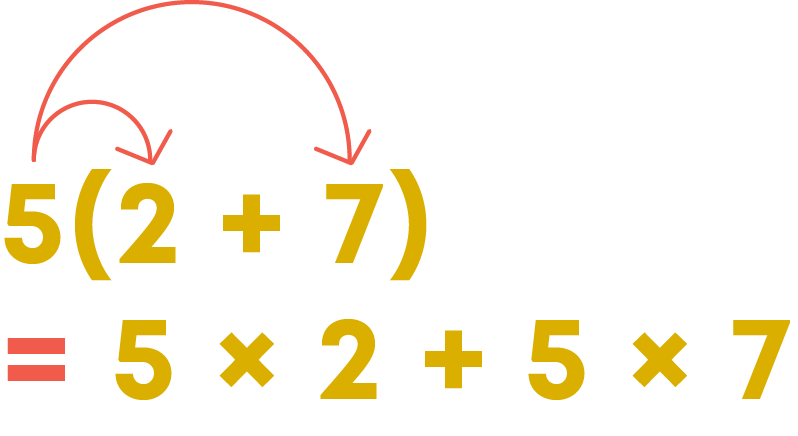Consider the multiplication of 2p by (3y + 1)

Can we apply distributive property here? Since variables take numerical values, they are also numbers. So, 2p and (3y + 1) are also numbers. Distributive property will be applicable for all numbers. So, we can apply it on the multiplication of 2p by (3y + 1).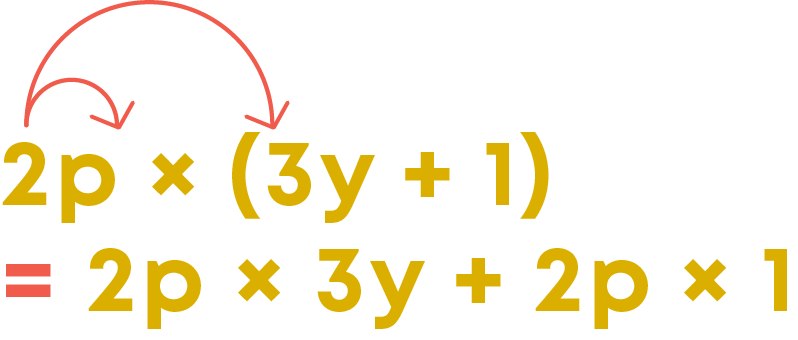Upon applying the property, the result will be: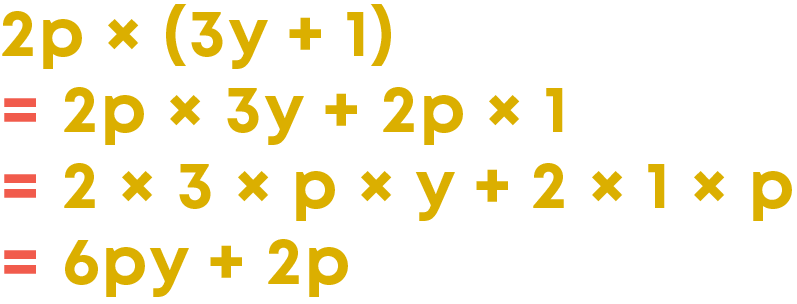To find the product of a monomial and a binomial,

• Multiply the monomial by each term of the binomial.
• Follow laws of exponents while multiplying variables.
• The product will be a binomial.

Now, apply distributive property on the multiplication of 2p and (2t – 3y + 1):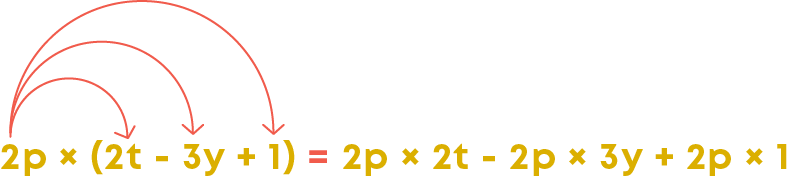How do you multiply each term of the trinomial (2t – 3y + 1) by the monomial 2p?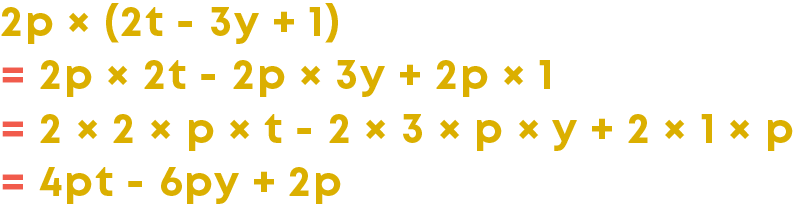To find the product of a monomial and a trinomial,

• Multiply the monomial by each term of the trinomial.
• Follow laws of exponents while multiplying variables.
• The product will be a trinomial.

To multiply a monomial by a polynomial, apply distributive property. Perform multiplication of the monomial by the first term of the polynomial, then by the second term and then by the third term and so on.

Multiplying A Polynomial By A Polynomial

Consider the multiplication of the binomial 3y + z by the binomial a – 2b:Suppose 3y + z is a single term, say p.

Now, how do you carry out the multiplication?

p(a – 2b) = p × a – p × 2b = pa – 2pb

Now replace p by 3y + z

(3y + z)(a – 2b) = (3y + z) × a - (3y + z) × 2b

Again, we get multiplication of a monomial and a binomial. Now, carry out the multiplication.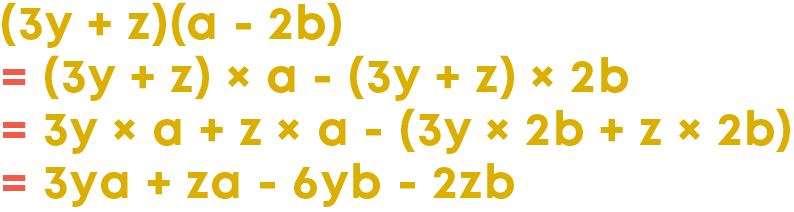So, by repeatedly applying distributive property we can multiply a binomial by a binomial.To find the product of two binomials,

• Multiply each term of one binomial by each term of the other binomial.
• Follow laws of exponents while multiplying variables.

Consider the multiplication of the binomial 3y + t by the trinomial a – 2b + cIn multiplication of a binomial by a binomial, we multiply each term of the second binomial by each term of the first binomial, one by one. Similarly, in the multiplication of a binomial by a trinomial, we multiply each term of the binomial by each term of the trinomial, one by one. Now, carry out the multiplication.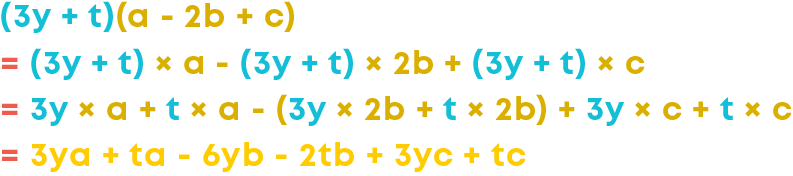So, by repeatedly applying distributive property we can multiply a binomial by a trinomial.

To find the product of a binomial and a trinomial,

• Multiply each term of one binomial by each term of the trinomial.
• Follow laws of exponents while multiplying variables.

To multiply a binomial by a polynomial, apply distributive property repeatedly. Multiply each term of the given binomial by each term of the given polynomial to get the product of a binomial and a polynomial.

#### Identity I: The Square Of A Binomial (a + b)

Is -2 a solution of the equation x + 1 = 0? No, -2 is not a solution of the equation. But what is the case of the equation (a + 1)2 = a2 + 2a + 1?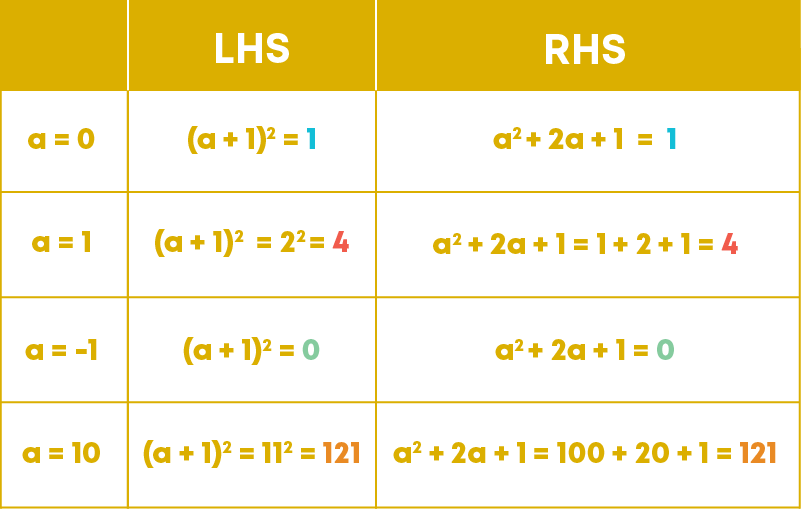0, 1, -1, and 10 are solutions of the equation (a + 1)2 = a2 + 2a + 1. Now, check whether 3 is a solution of the equation.

Substitute 3 in the place of ‘a’ on the LHS and the RHS separately and compare the values.3 also is a solution of the equation (a + 1)2 = a2 + 2a + 1.

Choose whichever number you want and check whether that number is a solution of the equation. You will find that any value of the variable is a solution of the equation. Thus, (a + 1)2 = a2 + 2a + 1 is an equality which is true for any value of the variable ‘a’. Such an equality is called an identity.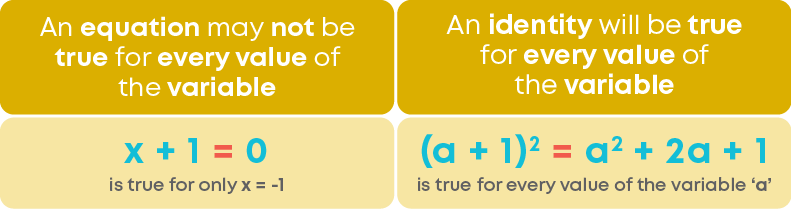An equation will not be true for every value of the variables. An identity will be true for every value of the variables.

You know how to multiply a binomial by a binomial. Now, multiply the binomial (a + b) by itself. What do you get?

If a number is multiplied by itself, what do you call the product? Its square. So, a + b multiplied by itself is (a + b)2.

So, (a + b)2 = (a + b)(a + b)

Now, apply the distributive property and multiply the binomial a + b by the binomial a + b.

(a + b)(a + b) = a2 + ab + ab + b2

= a2 + 2ab + b2

(a + b)2 = a2 + 2ab + b2 is a standard identity. It is visualised using rectangular and square pieces.

• (a + b)2 is the area of the largest square.
• a2 is the area of the larger square part.
• b2 is the area of the smaller square part.
• 2ab is the sum of the areas of the rectangular parts.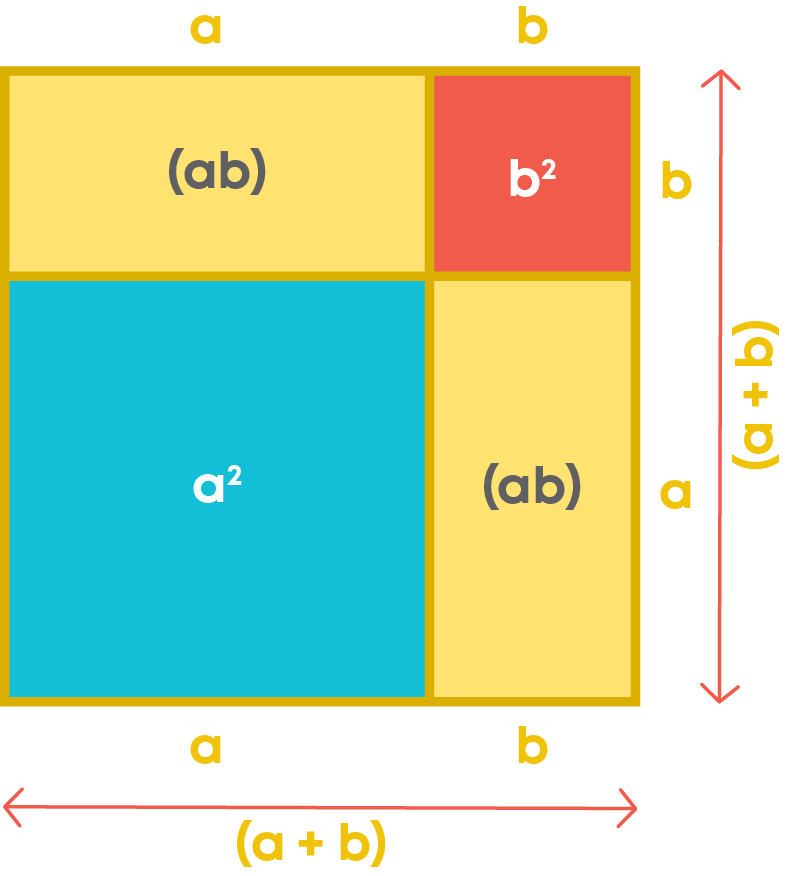You know that an identity is true for any value of the variables in it. Making use of this, we can find the square of other binomials. For example, substitute a = x and b = 1 in the identity (a + b)2 = a2 + 2ab + b2

What do you get?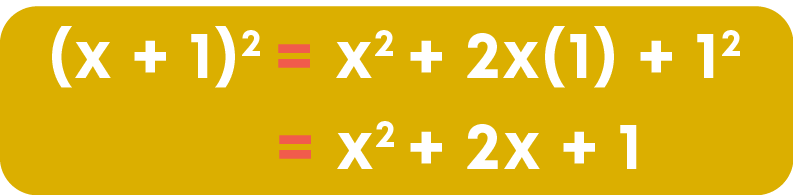So, here we found the square of the binomial x + 1 using the identity (a + b)2 = a2 + 2ab + b2

Making use of the identity, let us try to find the square of a large number, say 73.

How do you split 73 as the sum of two numbers? There are many ways of splitting it. But how do you know which is the best way of splitting it? Do not forget that we are making use of the identity to make the calculation easier. So, while choosing the way of splitting the number, keep it in mind that it should make the calculation easier. So, let us split 73 asNow, substitute a = 70 and b = 3 in the identity.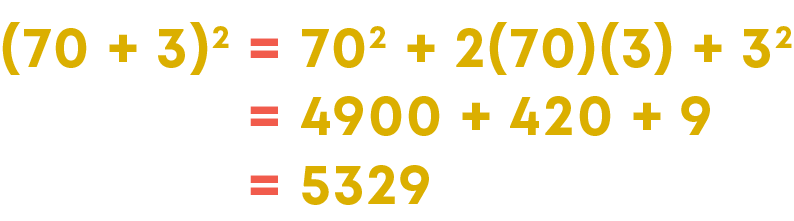#### Identity II: The Square Of A Binomial (a - b)

We know that an identity is true for every value of the variables in it. Since (a + b)2 = a2 + 2ab + b2 is an identity, it is true for any value of the variables ‘a’ and ‘b’. Now, if we substitute ‘-b’ in the place of ‘b’, will the equation be true? It must be true because (a + b)2 = a2 + 2ab + b2 is an identity. So, any value of ‘b’ is a solution of it. Let us substitute ‘-b’ in the place of ‘b’ and see what we get.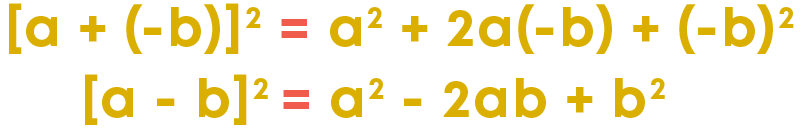What did we get? (a - b)2 = a2 - 2ab + b2

Let us verify this. What is (a – b)2? It is (a – b)(a – b). You know how to multiply a binomial by a binomial. Now, carry out the multiplication and check what you get.

(a – b)(a – b) = a2 – ab – ab + b2 = a2 - 2ab + b2

Thus, (a - b)2 = a2 - 2ab + b2 is also a standard identity. It is true for any value of the variables ‘a’ and ‘b.’

Making use of this, we can find the square of other binomials. For example, substitute a = x and b = 1 in the identity (a - b)2 = a2 - 2ab + b2. What do you get?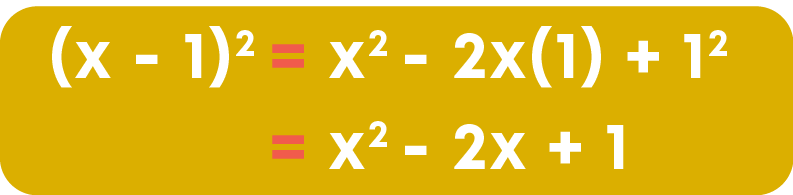Here we found the square of the binomial x - 1 using the identity (a - b)2 = a2 - 2ab + b2.

So, if you want to find the product of a binomial with itself, can we use this identity? Yes, finding the product of (a – b) and (a – b) is the same as finding the square of (a – b). For example, let us find the product of (x – 1) and (x – 1).

How do you find the product? Do you multiply (x – 1) by (x – 1) term by term? That can be done.

Also, since (x – 1) (x – 1) = (x – 1)2, we can use the identity (a - b)2 = a2 - 2ab + b2.

So, we have different ways of finding the product. Which one is the easier way? Let us compare.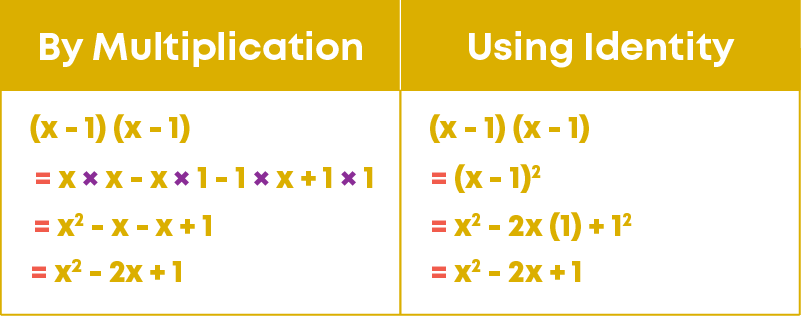The easier method to find the product is to use the identity (a - b)2 = a2 - 2ab + b2.

Making use of this, we can find the squares of numbers which will be lengthier to find by multiplication. For example, let us find the square of 67 using the identity. To apply the identity, 67 should be written as the difference of two numbers. How do you split 67 as the difference of two numbers? There are many ways of splitting it. But how do you know which is the best way of splitting it? We should choose the way that makes the calculation easier.

So, let us split 67 as 70 – 3. Now, substitute a = 70 and b = 3 in the identity (a - b)2 = a2 - 2ab + b2.

What do you get?

(70 – 3)2 = 702 – 2(70)(3) + 32

= 4900 – 420 + 9

= 4489

So, the square of 67 is 4489.

#### Identity III: The Difference Of Two Squares

Multiple the binomial (a + b) by (a – b). what do you get?(a + b)(a – b) = a2 – b2 is a standard identity.

To find the product of the form (a + b)(a – b), substitute the variables ‘a’ and ‘b’ by the corresponding terms of the given binomials in the identity (a + b)(a – b) = a2 – b2.

• Split the given two numbers as the sum and the difference of two numbers to get their product in the form (a + b)(a – b).
• Apply the identity (a + b)(a – b) = a2 – b2.
• Simplify.

To evaluate the expression of numbers given in the form a2 – b2:

• Convert the given expression of numbers to the form (a + b)(a – b) by applying the identity
• Simplify.

#### Identity IV: The Product Of Two Binomials Of The Form (x + a)(x + b)

How are the identities (a + b)2 = a2 + 2ab + b2 and (a - b)2 = a2 - 2ab + b2 obtained? By multiplying the binomials (a + b) and (a – b) by themselves respectively, we get those identities.

How do you obtain the identity (a + b)(a – b) = a2 – b2? By multiplying the binomial (a + b) by (a – b).

Now, multiply the binomial (x + a) by the binomial (x + b). What do you get?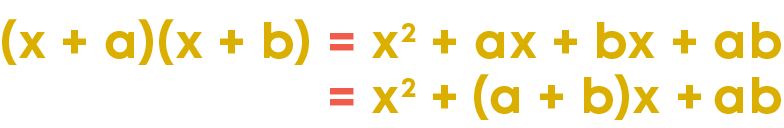Thus, (x + a)(x + b) = x2 + (a + b)x + ab is a standard identity.

The identity (x + a)(x + b) = x2 + (a + b)x + ab is a general form of the three standard identities: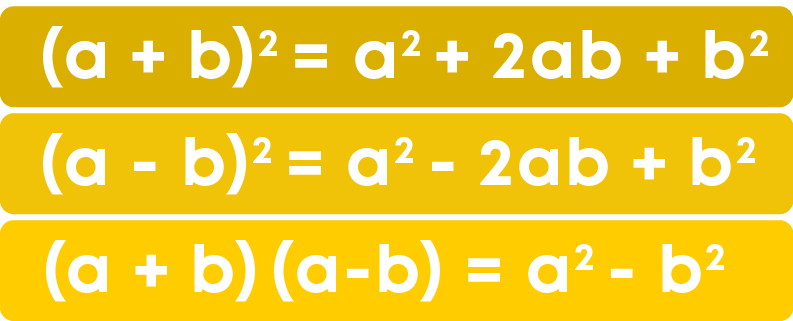To find the product of the form (x + a)(x + b), substitute the variables ‘a’ and ‘b’ by the corresponding terms of the given binomials in the identity (x + a)(x + b) = x2 + (a + b)x + ab.

Remember: the product of two numbers can be found by using the identity (x + a)(x + b) = x2 + (a + b)x + ab.

• Split each of the given numbers as the sum of two numbers such that one of the numbers in each are same, preferably a multiple of 10.
• Apply the identity and simplify.

We can simplify algebraic expressions using identities by Identifying the identity that can be applied on the given expression. More than one identity can be used to simplify an algebraic expression.

#### Common Errors

The following are topics in which students make common mistakes when dealing with algebraic expressions and identities:

• 1. Identifying terms of an expression
• 2. Operations on algebraic expressions
• 3. Identities
• 4. Multiplication of a monomial by a polynomial
• 5. Multiplication of a polynomial by a polynomial

#### Identifying Terms Of An Expression

While writing terms of an algebraic expression, the sign also should be considered. Example:

The terms of the expression x3 + 2x2 – 5x – 4 are x3, 2x2, 5x, and -4.

#### Operations On Algebraic Expressions

• While adding (or subtracting) terms with variables having exponents greater than 1, add (or subtract) only the coefficients. Do not add (or subtract) the exponents.
• While multiplying terms with common variables, add the exponents of common variables and multiply the coefficients.

Example: Add 4p3 and 7p3

4p3 + 7p3 = (4 + 7)p3 = 11p3

Multiply 4p3 by 7p3

4p3 × 7p3 = (4 × 7)p3+3 = 28p6

Multiply 4p3q2 by 7p

4p3q2 × 7p = (4 × 7)p3+1q2 = 28p4q2

#### Identities

The square of the sum of two numbers and the sum of the squares of two numbers are different.

Similarly, the square of the difference between two numbers and the difference between the squares of two numbers are different. If ‘a’ and ‘b’ are two numbers,

(a + b)2 ≠ a2 + b2

(a - b)2 ≠ a2 - b2

#### Multiplication Of A Monomial By A Polynomial

When we multiply a monomial by a polynomial or a polynomial by a monomial, make sure that each term of the polynomial is multiplied by the monomial (apply distributive property). Example: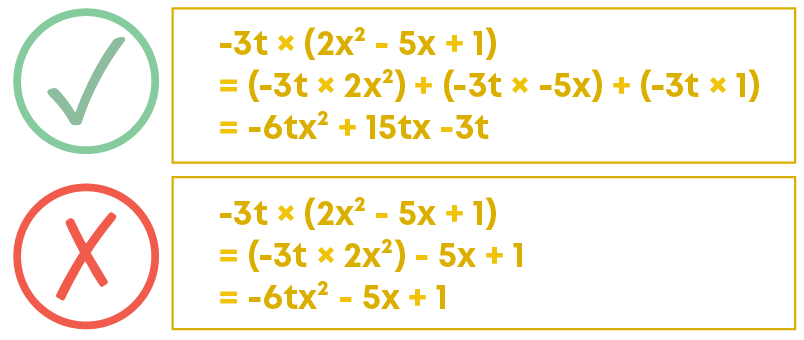#### Multiplication Of A Polynomial By A Polynomial

When we multiply a polynomial by a polynomial, make sure that each term of the first polynomial is multiplied by each term of the second polynomial (apply distributive property repetitively). Example: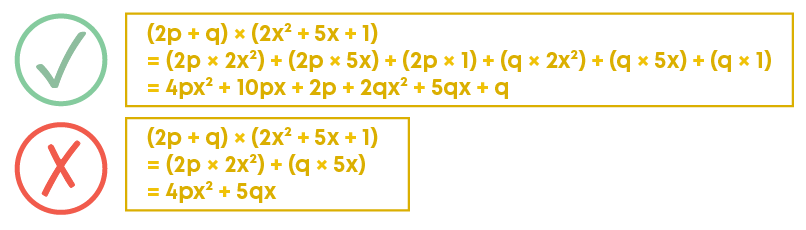#### Conclusion

You have learnt all about algebraic expressions and identities. Can you solve this riddle?

The areas of the rectangles within the big rectangle are given in the respective rectangles. What is the total area of the big rectangle?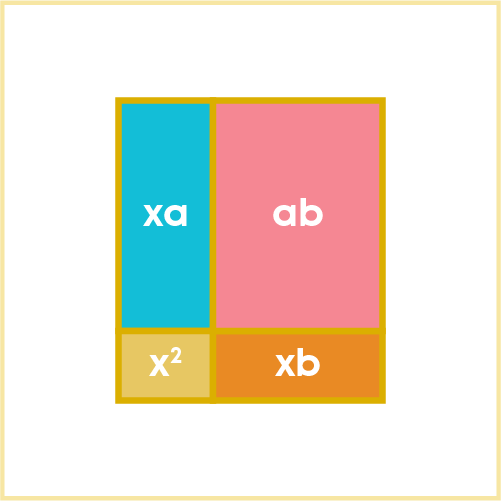#### Arpana

Author

Arpana is an education specialist with years of teaching Math and developing content. She previously worked as a freelance content developer, developing lesson plans for a reputed publisher of text books and content for various educational companies. She is an enthusiastic teacher who has taught students from India, the UK, and New Zealand.